$$\newcommand{\id}{\mathrm{id}}$$ $$\newcommand{\Span}{\mathrm{span}}$$ $$\newcommand{\kernel}{\mathrm{null}\,}$$ $$\newcommand{\range}{\mathrm{range}\,}$$ $$\newcommand{\RealPart}{\mathrm{Re}}$$ $$\newcommand{\ImaginaryPart}{\mathrm{Im}}$$ $$\newcommand{\Argument}{\mathrm{Arg}}$$ $$\newcommand{\norm}{\| #1 \|}$$ $$\newcommand{\inner}{\langle #1, #2 \rangle}$$ $$\newcommand{\Span}{\mathrm{span}}$$

# 6.R: Periodic Functions (Review)

•• Contributed by Jay Abramson
• Principal Lecturer (School of Mathematical and Statistical Sciences) at Arizona State University
• Sourced from OpenStax CNX

$$\newcommand{\vecs}{\overset { \rightharpoonup} {\mathbf{#1}} }$$

$$\newcommand{\vecd}{\overset{-\!-\!\rightharpoonup}{\vphantom{a}\smash {#1}}}$$

### 6.1: Graphs of the Sine and Cosine Functions

For the exercises 1-8, graph the functions for two periods and determine the amplitude or stretching factor, period, midline equation, and asymptotes.

1) $$f(x)=-3\cos x+3$$

amplitude: $$3$$; period: $$2\pi$$; midline: $$y=3$$$;$no asymptotes2) $$f(x)=\dfrac{1}{4}\sin x$$

3) $$f(x)=3\cos\left ( x+\dfrac{\pi }{6} \right )$$

amplitude: $$3$$; period: $$2\pi$$; midline: $$y=0$$; no asymptotes4) $$f(x)=-2\sin\left ( x-\dfrac{2\pi }{3} \right )$$

5) $$f(x)=3\sin\left ( x-\dfrac{\pi }{4} \right )-4$$

amplitude: $$3$$; period: $$2\pi$$; midline: $$y=-4$$; no asymptotes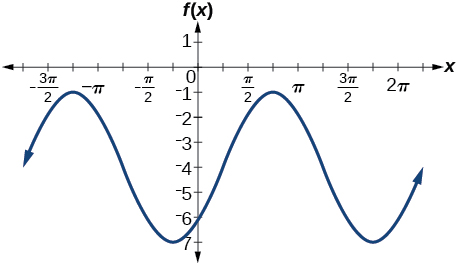6) $$f(x)=2\left (\cos\left ( x-\dfrac{4\pi }{3} \right )+1 \right )$$

7) $$f(x)=6\sin\left ( 3x-\dfrac{\pi }{6} \right )-1$$

amplitude: $$6$$; period: $$dfrac{2\pi }{3}$$; midline: $$y=-1$$; no asymptotes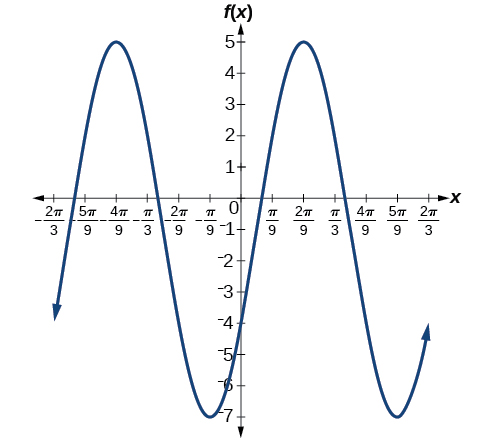8) $$f(x)=-100\sin(50x-20)$$

### 6.2: Graphs of the Other Trigonometric Functions

For the exercises 1-4, graph the functions for two periods and determine the amplitude or stretching factor, period, midline equation, and asymptotes.

1) $$f(x)=\tan x-4$$

stretching factor: none; period: $$\pi$$$;$midline: $$y=-4$$$;$asymptotes: $$x=\dfrac{\pi }{2}+\pi k$$, where $$k$$ is an integer2) $$f(x)=2\tan \left ( x-\dfrac{\pi }{6} \right )$$

3) $$f(x)=-3\tan (4x)-2$$

stretching factor: $$3$$; period: $$\dfrac{\pi }{4}$$; midline: $$y=-2$$; asymptotes: $$x=\dfrac{\pi }{8}+\dfrac{\pi }{4}k$$, where $$k$$ is an integer4) $$f(x)=0.2\cos(0.1x)+0.3$$

For the exercises 5-10, graph two full periods. Identify the period, the phase shift, the amplitude, and asymptotes.

5) $$f(x)=\dfrac{1}{3}\sec x$$

amplitude: none; period: $$2\pi$$; no phase shift; asymptotes: $$x=\dfrac{\pi }{2}k$$, where $$k$$ is an integer6) $$f(x)=3\cot x$$

7) $$f(x)=4\csc (5x)$$

amplitude: none; period: $$\dfrac{2\pi }{5}$$; no phase shift; asymptotes: $$x=\dfrac{\pi }{5}k$$, where $$k$$ is an integer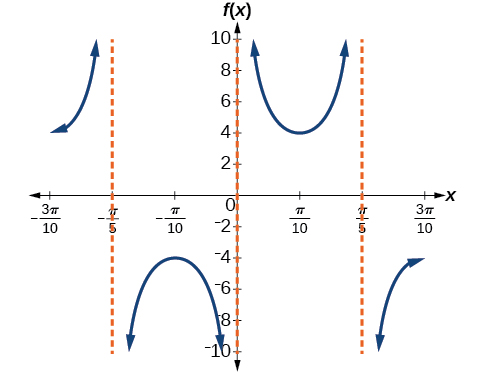8) $$f(x)=8\sec \left (\dfrac{1}{4}x \right )$$

9) $$f(x)=\dfrac{2}{3}\csc \left (\dfrac{1}{2}x \right )$$

amplitude: none; period: $$4\pi$$; no phase shift; asymptotes: $$x=2\pi k$$, where $$k$$ is an integer10) $$f(x)=-\csc (2x+\pi)$$

For the exercises 11-15, use this scenario: The population of a city has risen and fallen over a $$20$$-year interval. Its population may be modeled by the following function: $$y=12,000+8,000\sin(0.628x)$$, where the domain is the years since 1980 and the range is the population of the city.

11) What is the largest and smallest population the city may have?

largest: $$20,000$$; smallest: $$4,000$$

12) Graph the function on the domain of $$[0,40]$$.

13) What are the amplitude, period, and phase shift for the function?

amplitude: $$8,000$$; period: $$10$$; phase shift: $$0$$

14) Over this domain, when does the population reach $$18,000$$? $$13,000$$?

15) What is the predicted population in 2007? 2010?

In 2007, the predicted population is $$4,413$$. In 2010, the population will be $$11,924$$.

For the exercises 16a-16d, suppose a weight is attached to a spring and bobs up and down, exhibiting symmetry.

16) Suppose the graph of the displacement function is shown in the Figure below, where the values on the $$x$$-axis represent the time in seconds and the $$y$$-axis represents the displacement in inches.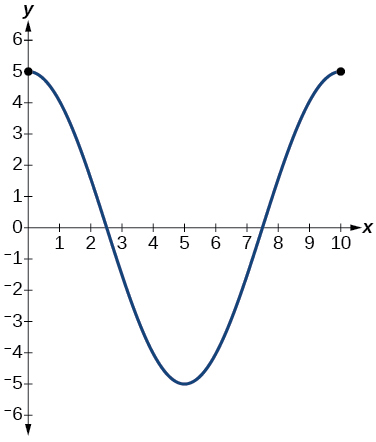1. Give the equation that models the vertical displacement of the weight on the spring.
2. At $$\text{time} = 0$$, what is the displacement of the weight?

$$5$$ in.

1. At what time does the displacement from the equilibrium point equal zero?
2. What is the time required for the weight to return to its initial height of $$5$$ inches? In other words, what is the period for the displacement function?

$$10$$ seconds

### 6.3: Inverse Trigonometric Functions

For the exercises 1-11, find the exact value without the aid of a calculator.

1) $$\sin ^{-1}(1)$$

2) $$\cos ^{-1}\left ( \dfrac{\sqrt{3}}{2} \right )$$

$$\dfrac{\pi }{6}$$

3) $$\tan ^{-1}(-1)$$

4) $$\cos ^{-1}\left ( \dfrac{1}{\sqrt{2}} \right )$$

$$\dfrac{\pi }{4}$$

5) $$\sin ^{-1}\left ( \dfrac{-\sqrt{3}}{2} \right )$$

6) $$\sin ^{-1}\left (\cos \left (\dfrac{\pi }{6} \right ) \right )$$

$$\dfrac{\pi }{3}$$

7) $$\cos ^{-1}\left (\tan \left (\dfrac{3\pi }{4} \right ) \right )$$

8) $$\sin \left (\sec^{-1} \left (\dfrac{3}{5} \right ) \right )$$

No solution

9) $$\cot \left (\sin^{-1} \left (\dfrac{3}{5} \right ) \right )$$

10) $$\tan \left (\cos^{-1} \left (\dfrac{5}{13} \right ) \right )$$

$$\dfrac{12}{5}$$

11) $$\sin \left (\cos^{-1} \left (\dfrac{x}{x+1} \right ) \right )$$

12) Graph $$f(x)=\cos x$$ and $$f(x)=\sec x$$ on the interval $$[0,2\pi )$$ and explain any observations.

The graphs are not symmetrical with respect to the line $$y=x$$.They are symmetrical with respect to the $$y$$-axis.13) Graph $$f(x)=\sin x$$ and $$f(x)=\csc x$$ and explain any observations.

14) Graph the function $$f(x)=\dfrac{x}{1}-\dfrac{x^3}{3!}+\dfrac{x^5}{5!}-\dfrac{x^7}{7!}$$ on the interval $$[-1,1]$$ and compare the graph to the graph of $$f(x)=\sin x$$ on the same interval. Describe any observations.

The graphs appear to be identical.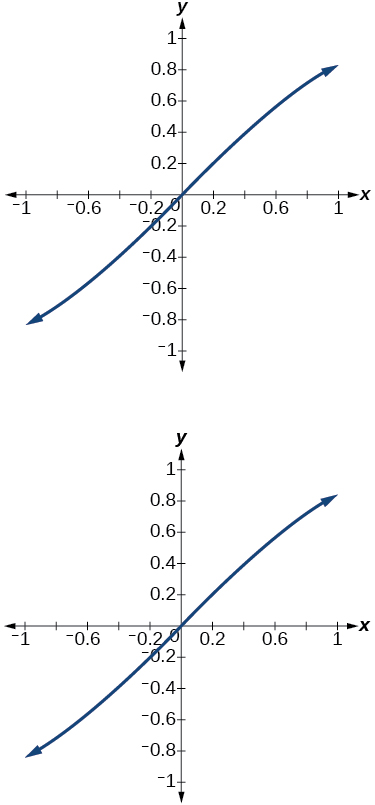### Practice Test

For the exercises 1-13, sketch the graph of each function for two full periods. Determine the amplitude, the period, and the equation for the midline.

1) $$f(x)=0.5\sin x$$

amplitude: $$0.5$$; period: $$2\pi$$$;$midline $$y=0$$

2) $$f(x)=5\cos x$$

3) $$f(x)=5\sin x$$

amplitude: $$0.5$$; period: $$2\pi$$; midline $$y=0$$4) $$f(x)=\sin (3x)$$

5) $$f(x)=-\cos \left ( x+\dfrac{\pi }{3} \right )+1$$

amplitude: $$1$$; period: $$2\pi$$; midline $$y=1$$6) $$f(x)=5\sin \left (3\left ( x-\dfrac{\pi }{6} \right ) \right )+4$$

7) $$f(x)=3\cos \left ( \dfrac{1}{3}x-\dfrac{5\pi }{6} \right )$$

amplitude: $$3$$; period: $$6\pi$$; midline $$y=0$$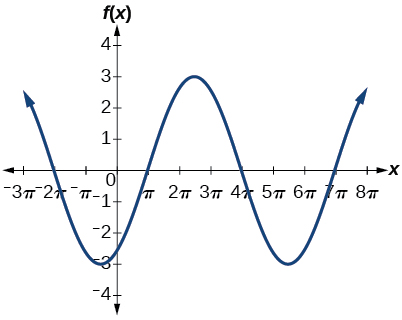8) $$f(x)=\tan (4x)$$

9) $$f(x)=-2\tan \left ( x-\dfrac{7\pi }{6} \right )+2$$

amplitude: none; period: $$\pi$$; midline $$y=0$$, asymptotes: $$x=\dfrac{2\pi }{3}+\pi k$$$,$ where $$k$$ is an integer10) $$f(x)=\pi \cos(3x+\pi)$$

11) $$f(x)=5\csc(3x)$$

amplitude: none; period: $$\dfrac{2\pi }{3}$$; midline $$y=0$$, asymptotes: $$x=\dfrac{\pi }{3}k$$$,$ where $$k$$ is an integer12) $$f(x)=\pi \sec \left ( \dfrac{\pi }{2}x \right )$$

13) $$f(x)=2\csc \left ( x+\dfrac{\pi }{4} \right )-3$$

amplitude: none; period: $$2\pi$$; midline $$y=-3$$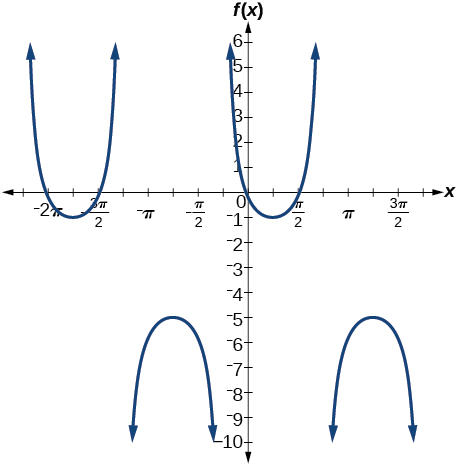For the exercises 14-16, determine the amplitude, period, and midline of the graph, and then find a formula for the function.

14) Give in terms of a sine function.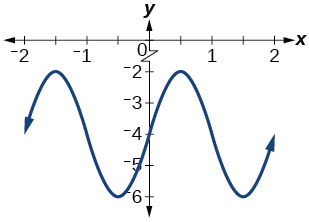15) Give in terms of a sine function.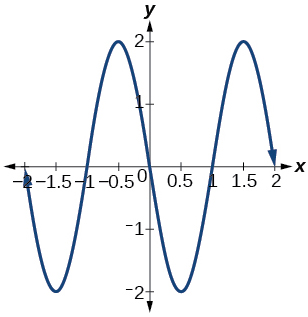amplitude: $$2$$; period: $$2$$; midline: $$y=0$$; $$f(x)=2\sin(\pi (x-1))$$

16) Give in terms of a tangent function.For the exercises 17-20, find the amplitude, period, phase shift, and midline.

17) $$y=\sin\left(\dfrac{\pi}{6}x+\pi \right)-3$$

amplitude: $$1$$; period: $$12$$; phase shift: $$-6$$; midline: $$y=-3$$

18) $$y=8\sin\left(\dfrac{7\pi}{6}x+\dfrac{7\pi}{2} \right)+6$$

19) The outside temperature over the course of a day can be modeled as a sinusoidal function. Suppose you know the temperature is $$68^{\circ}$$F at midnight and the high and low temperatures during the day are $$80^{\circ}$$F and $$56^{\circ}$$F, respectively. Assuming $$t$$ is the number of hours since midnight, find a function for the temperature, $$D$$, in terms of $$t$$.

$$D(t)=68-12\sin\left(\dfrac{\pi}{12}x \right)$$

20) Water is pumped into a storage bin and empties according to a periodic rate. The depth of the water is $$3$$ feet at its lowest at 2:00 a.m. and $$71$$ feet at its highest, which occurs every $$5$$ hours. Write a cosine function that models the depth of the water as a function of time, and then graph the function for one period.

For the exercises 21-25, find the period and horizontal shift of each function.

21) $$g(x)=3\tan(6x+42)$$

period: $$\dfrac{\pi}{6}$$; horizontal shift: $$-7$$

22) $$n(x)=4\csc \left(\dfrac{5\pi }{3}x-\dfrac{20\pi }{3} \right)$$

23) Write the equation for the graph in the Figure below in terms of the secant function and give the period and phase shift.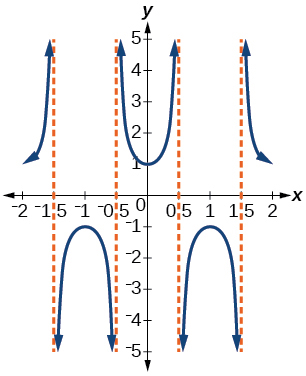$$f(x)=\sec(\pi x)$$; period: $$2$$; phase shift: $$0$$

24) If $$\tan x=3$$$,$find $$\tan (-x)$$.

25) If $$\sec x=4$$ ,   find $$\sec (-x)$$.

$$4$$

For the exercises 26-28, graph the functions on the specified window and answer the questions.

26) Graph $$m(x)=\sin(2x)+\cos(3x)$$ on the viewing window $$[-10,10]$$ by $$[-3,3]$$$.$Approximate the graph’s period.

27) Graph $$n(x)=0.02\sin(50\pi x)$$ on the following domains in $$x:[0,1]$$ and$$[0,3]$$$.$Suppose this function models sound waves. Why would these views look so different?

The views are different because the period of the wave is $$125$$$.$Over a bigger domain, there will be more cycles of the graph.28) Graph $$f(x)=\dfrac{\sin x}{x}$$ on $$[-0.5,0.5]$$ and explain any observations.

For the exercises 29-31, let $$f(x)=\dfrac{3}{5}\cos(6x)$$.

29) What is the largest possible value for $$f(x)$$?

$$\dfrac{3}{5}$$

30) What is the smallest possible value for $$f(x)$$?

31) Where is the function increasing on the interval $$[0,2\pi ]$$?

On the approximate intervals $$(0.5,1),(1.6,2.1),(2.6,3.1),(3.7,4.2),(4.7,5.2),(5.6,6.28)$$

For the exercises 32-33, find and graph one period of the periodic function with the given amplitude, period, and phase shift.

32) Sine curve with amplitude $$3$$, period $$\dfrac{\pi }{3}$$$,$and phase shift $$(h,k)=\left(\dfrac{\pi }{4},2\right)$$

33) Cosine curve with amplitude $$2$$, period $$\dfrac{\pi }{6}$$$,$and phase shift $$(h,k)=\left(-\dfrac{\pi }{4},3\right)$$

$$f(x)=2\cos\left ( 12\left ( x+\dfrac{\pi }{4} \right ) \right )+3$$For the exercises 34-35, graph the function. Describe the graph and, wherever applicable, any periodic behavior, amplitude, asymptotes, or undefined points.

34) $$f(x)=5\cos(3x)+4\sin(2x)$$

35) $$f(x)=e^{(sint)}$$

This graph is periodic with a period of $$2\pi$$For the exercises 36-43, find the exact value.

36) $$\sin^{-1}\left ( \dfrac{\sqrt{3}}{2} \right )$$

37) $$\tan^{-1}\left ( \sqrt{3} \right )$$

$$\dfrac{\pi }{3}$$

38) $$\cos^{-1}\left ( -\dfrac{\sqrt{3}}{2} \right )$$

39) $$\cos^{-1}\left ( \sin(\pi) \right )$$

$$\dfrac{\pi }{2}$$

40) $$\cos^{-1}\left ( \tan \left (\dfrac{7\pi}{4} \right ) \right )$$

41) $$\cos(\sin^{-1}(1-2x))$$

$$\sqrt{1-(1-2x)^2}$$

42) $$\cos^{-1}(-0.4)$$

43) $$\cos \left (\tan^{-1}\left(x^2\right) \right )$$

$$\dfrac{1}{\sqrt{1+x^4}}$$

For the exercises 44-46, suppose $$\sin t=\dfrac{x}{x+1}$$ $.$ Evaluate the following expressions.

44) $$\tan t$$

45) $$csc t$$

$$\dfrac{x+1}{x}$$

46) Given Figure, find the measure of angle $$\theta$$ to three decimal places. Answer in radians.For the exercises 47-49, determine whether the equation is true or false.

47) $$\arcsin\left(\sin\left(\dfrac{5\pi }{6}\right)\right)=\dfrac{5\pi }{6}$$

48) $$\arccos\left(\cos\left(\dfrac{5\pi }{6}\right)\right)=\dfrac{5\pi }{6}$$
49) The grade of a road is $$7\%$$. This means that for every horizontal distance of $$100$$ feet on the road, the vertical rise is $$7$$ feet. Find the angle the road makes with the horizontal in radians.
approximately $$0.07$$ radians